Chapter 4 Class 11 Mathematical Induction
Concept wise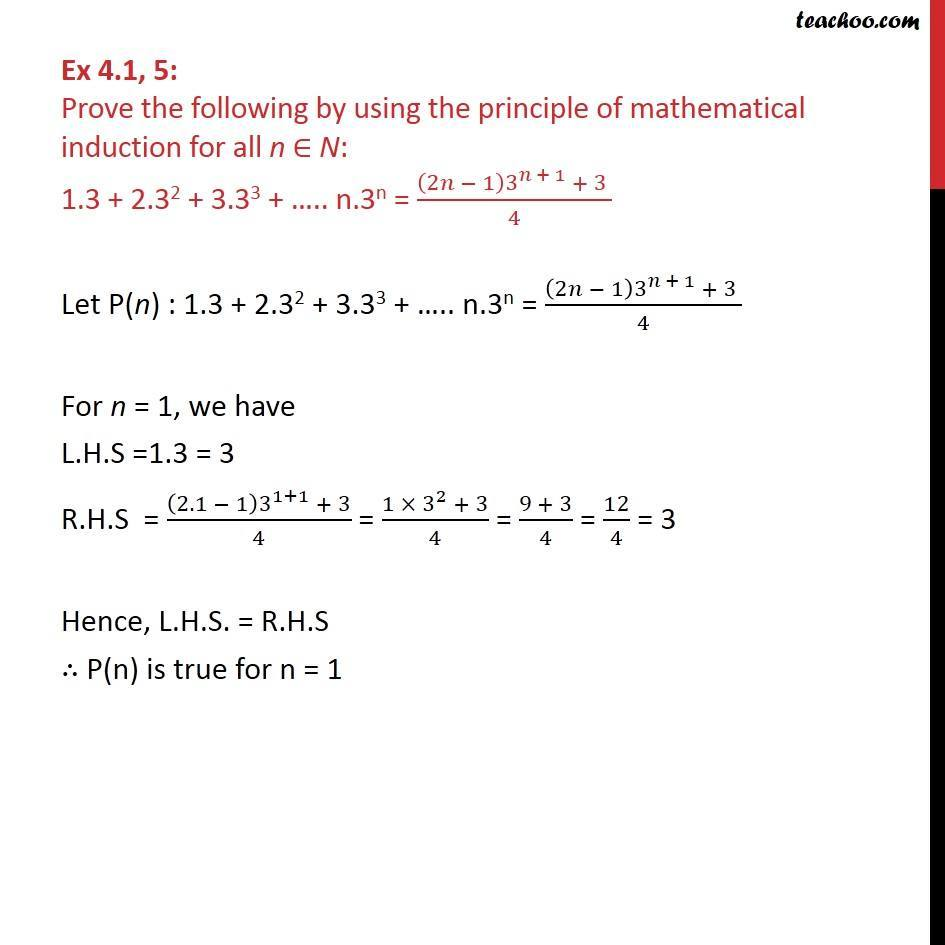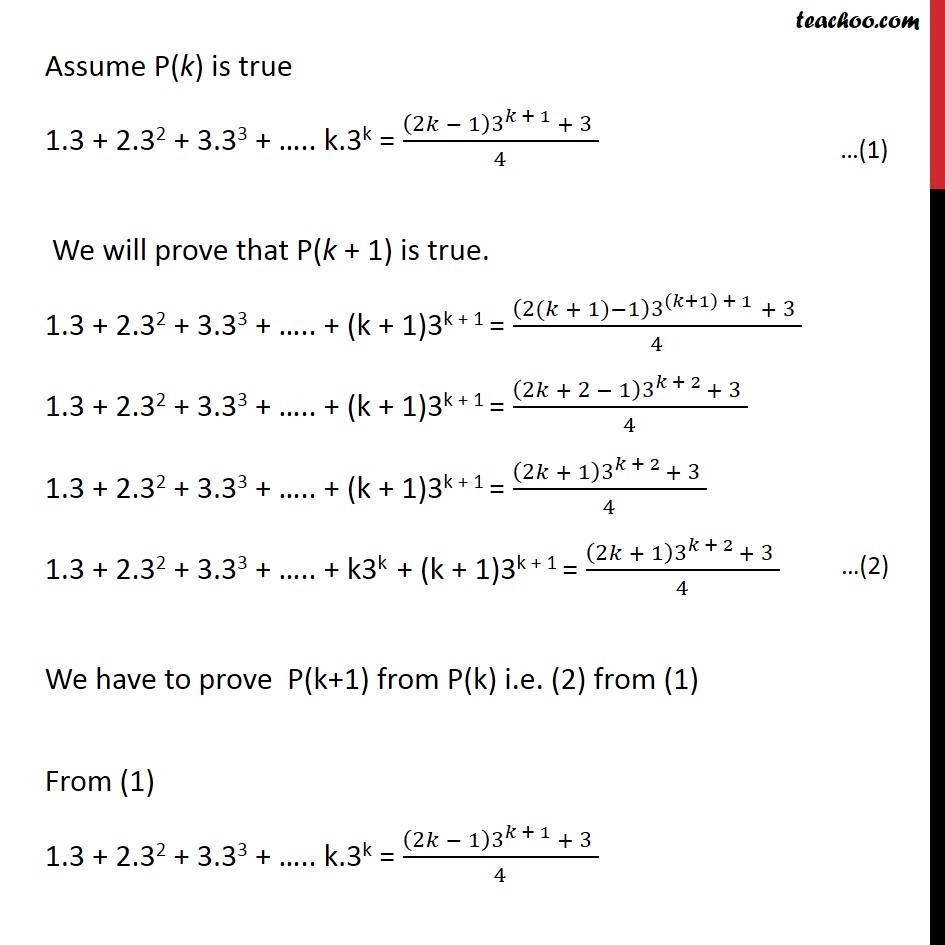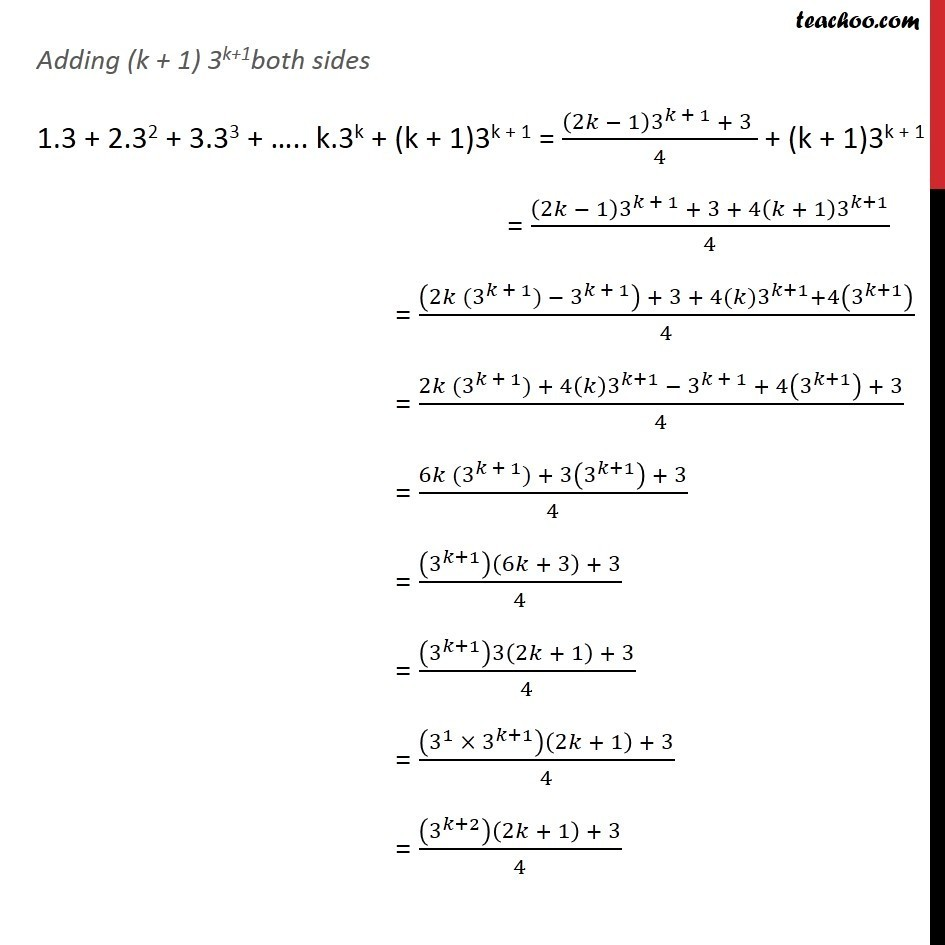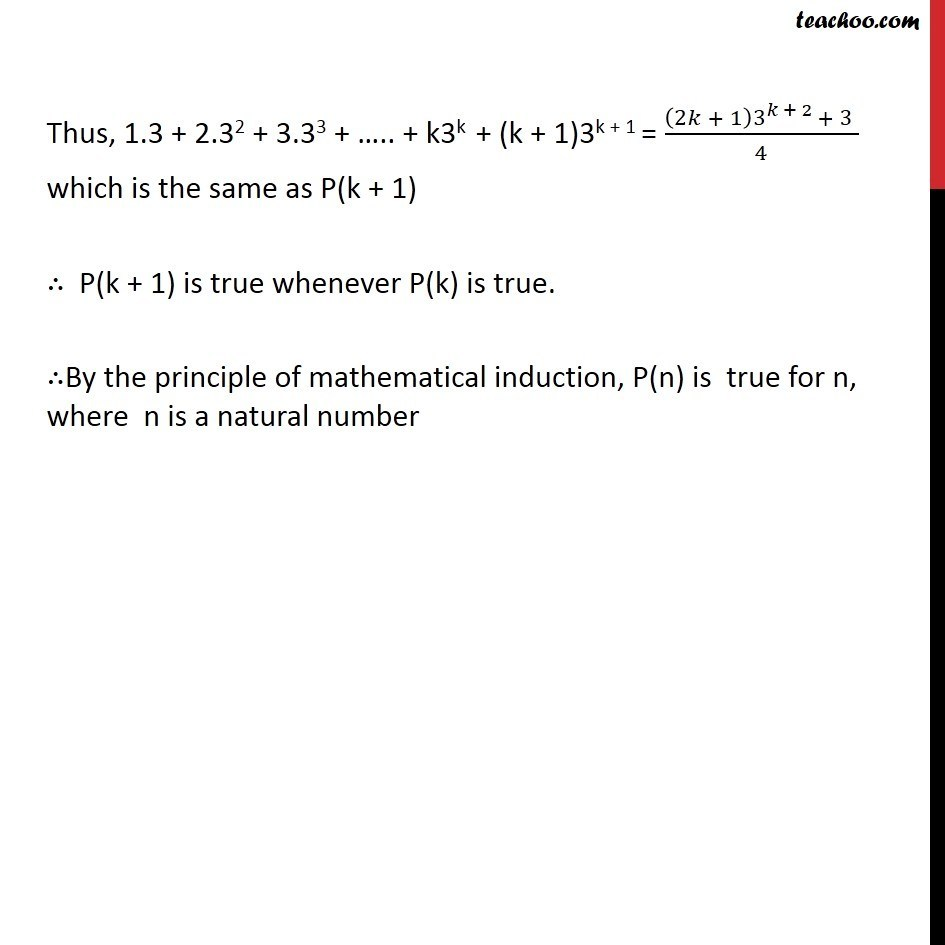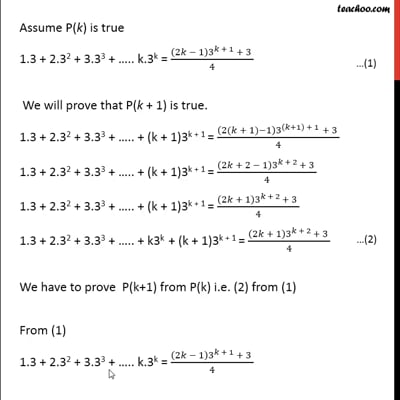This video is only available for Teachoo black users

Introducing your new favourite teacher - Teachoo Black, at only ₹83 per month

### Transcript

Ex 4.1, 5: Prove the following by using the principle of mathematical induction for all n N: 1.3 + 2.32 + 3.33 + .. n.3n = ((2 1) 3^( + 1) + 3 )/4 Let P(n) : 1.3 + 2.32 + 3.33 + .. n.3n = ((2 1) 3^( + 1) + 3 )/4 For n = 1, we have L.H.S =1.3 = 3 R.H.S = ((2.1 1) 3^(1+1) + 3)/4 = (1 3^2 + 3)/4 = (9 + 3)/4 = 12/4 = 3 Hence, L.H.S. = R.H.S P(n) is true for n = 1 Assume P(k) is true 1.3 + 2.32 + 3.33 + .. k.3k = ((2 1) 3^( + 1) + 3 )/4 We will prove that P(k + 1) is true. 1.3 + 2.32 + 3.33 + .. + (k + 1)3k + 1 = ((2( + 1) 1) 3^(( +1) + 1) + 3 )/4 1.3 + 2.32 + 3.33 + .. + (k + 1)3k + 1 = ((2 + 2 1) 3^( + 2) + 3 )/4 1.3 + 2.32 + 3.33 + .. + (k + 1)3k + 1 = ((2 + 1) 3^( + 2) + 3 )/4 1.3 + 2.32 + 3.33 + .. + k3k + (k + 1)3k + 1 = ((2 + 1) 3^( + 2) + 3 )/4 We have to prove P(k+1) from P(k) i.e. (2) from (1) From (1) 1.3 + 2.32 + 3.33 + .. k.3k = ((2 1) 3^( + 1) + 3 )/4 Adding (k + 1) 3k+1both sides 1.3 + 2.32 + 3.33 + .. k.3k + (k + 1)3k + 1 = ((2 1) 3^( + 1) + 3 )/4 + (k + 1)3k + 1 = ((2 1) 3^( + 1) + 3 + 4( + 1) 3^( +1))/4 = ((2 (3 ^( + 1)) 3^( + 1) ) + 3 + 4( )3^( +1)+4(3^( +1) ))/4 = (2 (3 ^( + 1)) + 4( ) 3^( +1) 3^( + 1) + 4(3^( +1) ) + 3)/4 = (6 (3 ^( + 1)) + 3(3^( +1) ) + 3)/4 = ((3^( +1) )(6 + 3) + 3)/4 = ((3^( +1) )3(2 + 1) + 3)/4 = ((3^1 3^( +1) )(2 + 1) + 3)/4 = ((3^( +2) )(2 + 1) + 3)/4 Thus, 1.3 + 2.32 + 3.33 + .. + k3k + (k + 1)3k + 1 = ((2 + 1) 3^( + 2) + 3 )/4 which is the same as P(k + 1) P(k + 1) is true whenever P(k) is true. By the principle of mathematical induction, P(n) is true for n, where n is a natural number